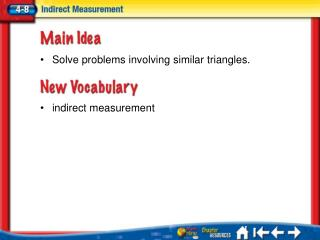# Lesson 8 MI/Vocab - PowerPoint PPT PresentationDownload PresentationLesson 8 MI/Vocab

Lesson 8 MI/VocabDownload Presentation## Lesson 8 MI/Vocab

- - - - - - - - - - - - - - - - - - - - - - - - - - - E N D - - - - - - - - - - - - - - - - - - - - - - - - - - -
##### Presentation Transcript

1. Solve problems involving similar triangles. • indirect measurement Lesson 8 MI/Vocab

2. 8.3The student identifies proportional or non-proportional linear relationships in problem situations and solves problems. (B)Estimate and find solutions to application problems involving percents and other proportional relationships such as similarity and rates. 8.9The student uses indirect measurement to solve problems.(B)Use proportional relationships in similar two-dimensional figures or similar three-dimensional figures to find missing measurements. Lesson 8 TEKS

3. Use Shadow Reckoning TREES A tree in front of Marcel’s house has a shadow 12 feet long. Marcel’s shadow is 3 feet long. If Marcel is 5.5 feet tall, how tall is the tree? Lesson 8 Ex1

4. tree’s shadow tree’s height Marcel’s shadow Marcel’s height Use Shadow Reckoning 12 ● 5.5 = 3 ● h Find the cross products. Multiply. Divide each side by 3. Simplify. Answer: The tree is 22 feet tall. Lesson 8 Ex1

5. Jayson casts a shadow that is 10 feet long. At the same time, a flagpole casts a shadow that is 40 feet long. If the flagpole is 20 feet tall, how tall is Jayson? • A • B • C • D A. 4.5 feet B. 5 feet C. 5.5 feet D. 6 feet Lesson 8 CYP1

6. Use Indirect Measurement SURVEYING The two triangles shown in the figure are similar. Find the distance d across the stream. Lesson 8 Ex2

7. Use Indirect Measurement Write a proportion. AB= 48, ED=d, BC = 60, and DC = 20 48 ● 20 = d ● 60 Find the cross products. Multiply. Then divide each side by 60. Simplify. Answer: The distance across the stream is 16 meters. Lesson 8 Ex2

8. SURVEYINGThe two triangles shown in the figure are similar. Find the distance d across the stream. • A • B • C • D A. 6 feet B. 6.5 feet C. 7 feet D. 7.5 feet Lesson 8 CYP2